### Home > PC > Chapter 2 > Lesson 2.3.1 > Problem2-73

2-73.
1. Lew walks every day at lunch when he is working. The amazing thing about Lew is that he walks at a constant rate (velocity) for the whole time he walks. He walks for 1 hour every day and travels at a rate of 4 miles per hour. Homework Help ✎

1. Draw a graph of Lew’s lunchtime walk, being sure to label both axes. Shade the area between the curve f(x) and the x­-axis over the appropriate domain.

2. Explain why the domain will only be between 0 and 1. What is the range of this function?

3. The figure that is shaded on your graph should resemble a rectangle. Using unit analysis and the units from each axis, find the resulting units when you find the area of the rectangle.

4. Find the area of the shaded region. Include the proper units.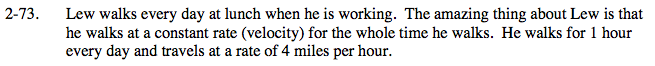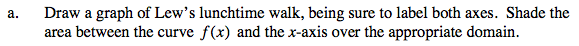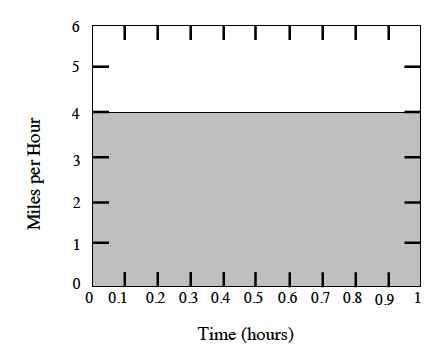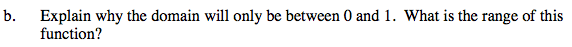The domain is time elapsed, and Lew walks for one hour. The range is 4 miles per hour.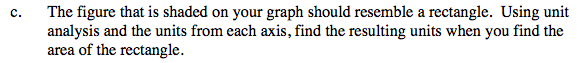The units are miles since:

$\frac{\text{miles}}{\text{hour}}\cdot \text{hr}=\text{miles}$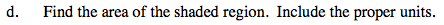Use the graph above to figure this out.